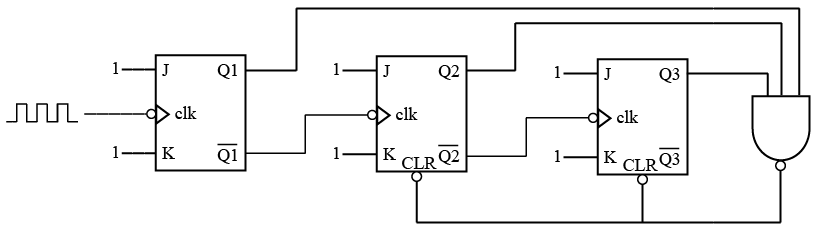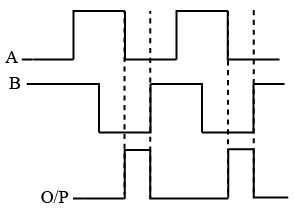# ISRO Scientist or Engineer Electronics 2016

Instructions

For the following questions answer them individually

Question 1

# The approximate O/P Frequencyof 555 Oscillator for $$R_A = R_b = 2.2 kΩ$$ and C = 2000 pFQuestion 2

# A counter is designed using J-K Flip-Flop as shown in fig. Define its count sequenceQuestion 3

# A 1 MHz clock signal is applied to a J-K Flip Flop with J = K = 1. What is the frequency of the Flip-Flop O/P signal?Question 4

# How many inputs & outputs does a full adder have?Question 5

# Which shift register counter requires the most decoding circuitry?Question 6

# A 10-bit DAC hasa step size of 10mV. What is its Full scale O/P voltage and the percentage resolution?Question 7

# If the input signals (A & B) and output signals are as below then the circuit element isQuestion 8

# For a 10-bit digital ramp ADC using 500kHz clock, the maximum conversion time isQuestion 9

# In Digital Filters, how many interpolated data points are inserted between samples when performing 4X over sampling?Question 10

# A PAM source generates four symbols 3 V, 1 V, -1 V and —3 V with probability of p(3) = 0.2, p(1) = 0.3, p(-1) = 0.3, p(—3) = 0.2 respectively. The variance for this source will beOR